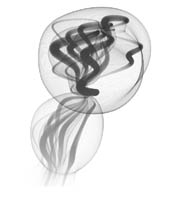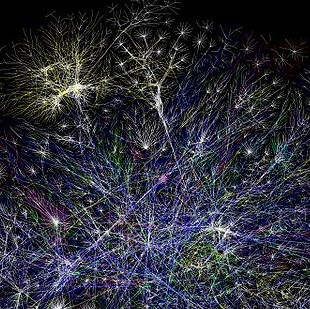Notes from Jan 26, 2015, CS110 Introduction to Computing, Bryn Mawr College. Douglas Blank.

# 1. What can you do with Processing?¶

Some examples:Now, let's see what you can do! Assignment #1

# 2. Jupyter and Processing¶

## 2.1 Jupyter¶

Jupyter is the name of the system that you log into. It is running on a server at Bryn Mawr College. It allows you to run code in a variety of programming languages inside things called "notebooks." Each notebook is made up of cells. Cells can have text (called "markdown", like this text).

You can turn on spell-checking by clicking the button in the toolbar.

You can control how a cell is treated (code or markdown) by selecting from the picklist in the toolbar.

You can also put code in a cell, like this:

In :
fill(0);
text("This is a sketch", 10, 15);
text("You can write", 10, 30);
text("and draw:", 10, 45);
fill(255, 0, 0);
ellipse(50, 75, 25, 25);

Sketch #11:

Cells should be run sequentially, from top to bottom. You can get information on how to use the notebook for writing here: Jupyter Notebook Users Manual.

Tips:

• You can press SHIFT+TAB after a name to get help on it
• you can press TAB after a few letters to complete the variable or function

You can create text with headings, styles, and even create equations, like:

$$\left(\! \begin{array}{c} n \\ r \end{array} \!\right) = \frac{n!}{r!(n-r)!}$$

## 2.2 Processing¶

Processing is a programming language. You write programs as "sketches." Each sketch goes into a Jupyter cell.

The syntax of the Processing language is a subset of the Java programming language. After you write a sketch (a program) in Processing, there are a number of things that you can do with it:

1. compile it and run it with Java
2. translate it to JavaScript and run it

We are doing number 2 here. Note that there are some differences between these two methods:

• The Java version can use Java libraries (like robot control)
• The Java version can create files, and do things on your computer

# 3. Sketch¶

There are two special functions: draw() and setup():

• put code in setup() that is to be run once, at the start of your program.
• put code in draw() that is to be run many times a second

You don't have to use those two methods. For now, you can simply put code in the sketch outside of any function.

Functions to explore:

• size()
• background()
• point()
• line()
• triangle()
• rectangle()
• ellipse()

# 4. Draw by Numbers (DBN)¶

Here we will draw a circle in the draw() function by using the ellipse function.

What is a function?

In :
void draw() {
ellipse(50, 50, 80, 80);
}

Sketch #1:

We can make the canvas larger by using the size() function inside the setup() function. size takes a width and a hight as arguments.

In :
void setup() {
size(500, 500);
}

void draw() {
ellipse(50, 50, 80, 80);
}

Sketch #2:

It would be nice to make the circle draw in the center with a size proportional to the size of the canvas.

In :
int width = 500;
int height = 500;

void setup() {
size(width, height);
}

void draw() {
ellipse(width/2, height/2, width, height);
}

Sketch #14: